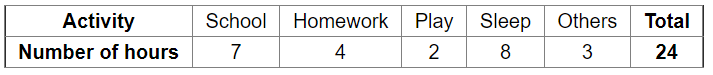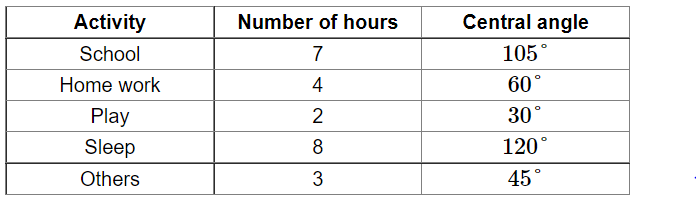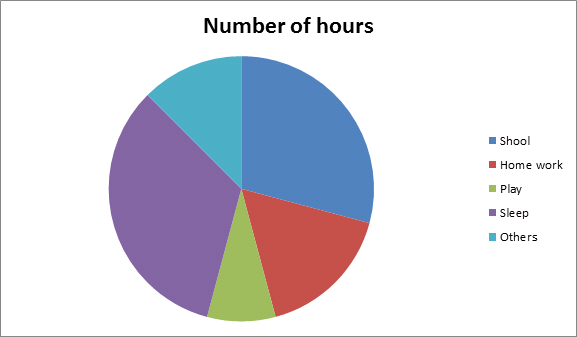# The data given below shows number of hours spent by a school boy on different activities on a working day.

Question:

The data given below shows number of hours spent by a school boy on different activities on a working day.Represent the above data by a pie chart.

Solution:

Total number of hours $=24$

Central angle of each component $=\left(\frac{\text { number of hours spent on each activity }}{\text { total number of hours }} \times 360\right)^{\circ}$

Calculation of central anglesConstruction of pie chart

Steps of construction:

1. Draw a circle of any convenient radius.

2. Draw a horizontal radius of this circle.

3. Draw sectors whose central angles are $105^{\circ}, 60^{\circ}, 30^{\circ}, 120^{\circ}$ and $45^{\circ}$.

4. Shade the sectors so obtained differently and label each one of them.

Thus, we obtain the required pie chart as shown in the figure below.